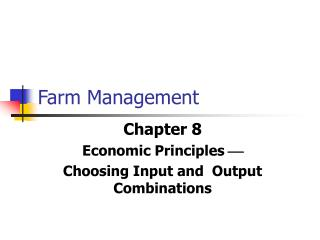DownloadDownload PresentationFarm Management

# Farm Management

Download Presentation## Farm Management

- - - - - - - - - - - - - - - - - - - - - - - - - - - E N D - - - - - - - - - - - - - - - - - - - - - - - - - - -
##### Presentation Transcript

1. Farm Management Chapter 8 Economic Principles  Choosing Input and Output Combinations

2. Chapter Outline • Input Combinations • Enterprise Combinations

3. Input Combinations Most products require two or more inputs, and the manager may choose the input combination or ratio to use. The economic question is whether one input can be substituted for another to reduce the cost.

4. Types of Input Substitution • Constant rate (perfect substitution) • Decreasing rate • No substitution

5. Choosing Inputs • We will address how to minimize cost for a given level of output. • We will do so by combining isocosts with isoquants

6. Q1is an isoquant for output Q1. Isocost curve C0 shows all combinations of K and L that can produce Q1at this cost level. K2 CO C1 C2 are three isocost lines A K1 Q1 K3 C0 C1 C2 L2 L1 L3 Producing a GivenOutput at Minimum Cost Capital per year Isocost C2 shows quantity Q1 can be produced with combination K2L2or K3L3. However, both of these are higher cost combinations than K1L1. Labor per year

7. If the price of labor changes, the isocost curve becomes steeper due to the change in the slope -(w/L). This yields a new combination of K and L to produce Q1. Combination B is used in place of combination A. The new combination represents the higher cost of labor relative to capital and therefore capital is substituted for labor. B K2 A K1 Q1 C2 C1 L2 L1 Input Substitution When an Input Price Change Capital per year Labor per year

8. Cost in the Long Run • Isoquants and Isocosts and the Production Function

9. Input Substitution Ratio Input substitution ratio = amount of input replaced amount of input added

10. The expansion path illustrates the least-cost combinations of labor and capital that can be used to produce each level of output in the long-run. \$3000 Isocost Line Expansion Path \$2000 Isocost Line C B 300 Unit Isoquant A 200 Unit Isoquant A Firm’s Expansion Path Capital per year 150 100 75 50 25 Labor per year 100 150 200 300 50

11. Expansion Path F E D A Firm’s Long-Run Total Cost Curve Cost per Year 3000 2000 1000 Output, Units/yr 100 200 300

12. Figure 8-1Three possible types of substitution

13. Input Price Ratio Input price ratio = price of input being added price of input being replaced

14. Decision Rule input substitution ratio = input price ratio If they cannot be exactly equal because of the choices available in the table, get as close as possible without letting the price ratio exceed the substitution ratio.

15. Table 8-1 Selecting a Least-Cost Feed Ration grain at 4.4¢ and hay at 3.0¢

16. With Different Types of Substitution • With a constant rate of substitution, the least-cost combination will be all of one input and none of the other (unless the price ratio is exactly equal to the constant rate of substitution). • With a decreasing rate of substitution, the least-cost combination will usually include some of each input.

17. Enterprise Combinations Another decision that must be made is the combination of enterprises to produce to maximize profits. If one or more inputs is limited, there is an upper limit on how much can be produced.

18. Enterprise Relationships The first step in determining the profit-maximizing combination of enterprises is to determine the physical relationship among the enterprises.

19. Types of Relationships • Competitive: output of one enterprise cannot be increased unless output of the other decreases • Supplementary: more output from one enterprise can be added without a change in the level of the other enterprise • Complementary: as output of one enterprise increases, output of the other increases also

20. Figure 8-3 Supplementary & complementary enterprise relationships

21. Each curve shows combinations of output with a given combination of L,L & K. O2 O1 illustrates a low level of output. O2 illustrates a higher level of output with two times as much labor, land and capital. O1 Product Transformation Curve Corn Soybeans

22. Production with TwoOutputs--Economies of Scope • Observations • Product transformation curves are negatively sloped • Constant returns exist in this example

23. Output Substitution Ratio Output Substitution Ratio = quantity of output lost quantity of output gained

24. Output Price Ratio Output Price Ratio = price of output gained price of output lost

25. Decision Rule output substitution ratio = output price ratio If no available combination makes these exactly equal, get as close as possible without letting the price ratio drop below the substitution ratio.

26. Table 8-2Profit-Maximizing Enterprise Combination corn at \$2.80/bu, wheat at \$4.00/bu

27. Summary This chapter emphasizes the use of substitution principles to decide how and what to produce. To decide how to produce, the manager finds the least-cost combination of inputs. To decide what to produce, the manager finds the profit-maximizing combination of enterprises.